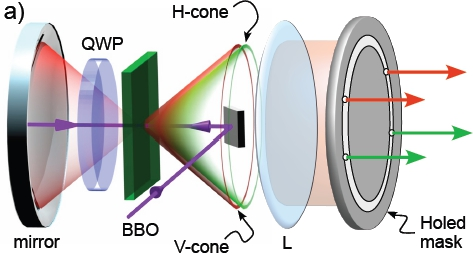Entanglement is the most distinctive feature of quantum physics and represents the key resource of Quantum Information (QI). By using entanglement we can perform computational and cryptographic tasks, otherwise impossible with classical systems, and also investigate the nonlocal properties of the quantum world. Quantum photon states are currently generated and accurately manipulated using linear and nonlinear optical devices and measured by efficient single-photon detectors. Photonic qubits represent the typical QI carrier and can be distributed over long distances, either in free-space or in low-loss optical fibers.
A large number of qubits is generally required to simulate complex quantum systems, to realize quantum algorithms of increasing complexity and to investigate quantum world at a mesoscopic level.Two approaches may be envisaged in this direction. The first one consists of increasing the number of entangled particles. In this way, multi-qubit entangled states are created by distributing the qubits between the particles so that each particle carries one qubit.
A second strategy consists of encoding more than one qubit in each particle, by exploiting differentdegrees of freedom (DOFs) of the photon such as the polarization, the path, the emission time. Hyperentanglement corresponds exactly to entangle two particles in different DOFs.
The most important experiments dealing with hyperentangled states of two photons are:
1. The generation of particular families of high-dimensional multi-qubit quantum states. In fact, by manipulating the hyperentangled states it has been possible to engineer optical sources for 4- and 6-qubit Cluster states  and for 4-qubit Phased Dicke states . While the former have been used to implement QI protocols [1,4], by the second type of states it was possible to study the resilience of entanglement to the noise .

2. The realization of a versatile source for 2-qubit mixed states, obtained by tracing out the path DOF in the 4-qubit HE state. This source has been used to study extremal quantum correlations by using quantum discord .

3. The first experimental implementation of an optimal protocol for the characterization of a single-qubit noisy channel .
Next development deals with three main topics:
1. Experimental study of Non-Markovian dynamics based on low-dimensional quantum states (i.e. 3-qubit)

2. Development of higher-dimension quantum photon states (up to 8-qubit)

3. Miniaturization of the existing bulk experiments by exploiting the quantum integrated circuits.